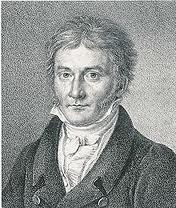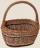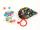# Gauss

Help little C.F. Gauss sum all the integers from 1 to 400.

Correct result:

s =  80200

#### Solution:We would be pleased if you find an error in the word problem, spelling mistakes, or inaccuracies and send it to us. Thank you!## Next similar math problems:

• Sequence 3Write the first 5 members of an arithmetic sequence: a4=-35, a11=-105.
• SeatsSeats in the sport hall are organized so that each subsequent row has five more seats. First has 10 seats. How many seats are: a) in the eighth row b) in the eighteenth row
• AS sequenceIn an arithmetic sequence is given the difference d = -3 and a71 = 455. a) Determine the value of a62 b) Determine the sum of 71 members.In six baskets, the seller has fruit. In individual baskets, there are only apples or just pears with the following number of fruits: 5,6,12,14,23 and 29. "If I sell this basket," the salesman thinks, "then I will have just as many apples as a pear." Whic
• SequenceWrite the first 7 members of an arithmetic sequence: a1=-3, d=6.
• SequenceWrite the first 6 members of these sequence: a1 = 5 a2 = 7 an+2 = an+1 +2 an
• What is 9What is the 15th term; x1=1.5, d=4.5?
• TreesA certain species of tree grows an average of 0.5 cm per week. Write an equation for the sequence that represents the weekly height of this tree in centimeters if the measurements begin when the tree is 200 centimeters tall.
• AP - simpleDetermine the first nine elements of sequence if a10 = -1 and d = 4
• SequenceBetween numbers 1 and 53 insert n members of the arithmetic sequence that its sum is 702.Determine how many apples are in baskets when in the first basket are 4 apples, and in any other is 29 apples more than the previous, and we have eight baskets.Michal said to Martin: give me one ball and I'll have twice as you. Martin said: give me 4 and we will have equally. How many balls each have?Find the first six terms of the sequence. a1 = 7, an = an-1 + 6Write the first 5 members of an arithmetic sequence a11=-14, d=-1What is the 10th term of the Arithmetic Progression if x1=4 and d=5?Find the nineteenth member of the arithmetic sequence: a1=33 d=5 find a19One number is 2 less than a second number. Twice the second number is 14 less than 5 times the first. Find the two numbers.